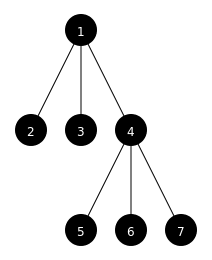This is an alternative site for discovering Elm packages. You may be looking for the official Elm package site instead.
Library for drawing diagrams of trees
 version 3.0.0 license BSD3 native-modules False elm-version 0.18.0 <= v < 0.19.0
 Tag 3.0.0 Committed At 2017-02-19 04:01:19 UTC
 evancz/elm-graphics 1.0.1 <= v < 2.0.0 1.0.1 elm-lang/svg 2.0.0 <= v < 3.0.0 2.0.0 elm-lang/html 2.0.0 <= v < 3.0.0 2.0.0 elm-lang/core 5.1.1 <= v < 6.0.0 5.1.1

# elm-tree-diagram

This is an Elm package for drawing diagrams of trees. For positioning the trees it uses the approach described in Tidier Drawings of Trees.

## Usage

Here's the tree data structure we want to draw:

``````coolTree =
node
1
[ node 2 []
, node 3 []
, node
4
[ node 5 []
, node 6 []
, node 7 []
]
]
``````

We first define a function for drawing the tree nodes, which in this case each contain an integer. We'll display each node as white text on a black circle.

``````drawNode n =
group
[ circle 16 |> filled black
, toString n |> fromString |> Text.color white |> text
]
``````

Then we define a function for drawing the edges between nodes.

``````drawEdge to =
segment (0, 0) to |> traced (solid black)
``````

Finally we call draw with our tree, node drawer, and edge drawer:

``````main =
toHtml <|
draw defaultTreeLayout drawNode drawEdge coolTree
``````

This should produce the diagram below.The argument `defaultTreeLayout` contains some options for configuring the layout of the tree. See the API section below for more details.

## API

### TreeDiagram.Canvas

``````draw : TreeLayout -> NodeDrawer a Form -> EdgeDrawer Form -> Tree a -> Element
``````

Draws the tree as a canvas image using the provided functions for drawings nodes and edges.

### TreeDiagram.Svg

``````draw : TreeLayout -> NodeDrawer a (Svg msg) -> EdgeDrawer (Svg msg) -> Tree a -> Html msg
``````

Draws the tree as an SVG using the provided functions for drawings nodes and edges.

### TreeDiagram

``````node : a -> List (Tree a) -> Tree a
``````

Creates a tree of type `a` from a node value and a list of subtrees.

``````type alias NodeDrawer a format = a -> format
``````

Alias for functions that draw nodes of type `a` in the given `format` (e.g. `Svg msg` or `Form`).

``````type alias EdgeDrawer format = Coord -> format
``````

Alias for functions that draw edges between nodes in the given `format`.

``````type alias TreeLayout =
{ orientation : TreeOrientation
, levelHeight : Int
, subtreeDistance : Int
, siblingDistance : Int
}
``````

Options for laying out the tree:

• orientation: direction of the tree from root to leaves.
• levelHeight: vertical distance between parent and child nodes.
• subtreeDistance: horizontal distance between subtrees.
• siblingDistance: horizontal distance between siblings. This is usually set below `subtreeDistance` to produce a clearer distinction between sibling nodes and non-siblings on the same level of the tree.
• padding: amount of space to leave around the edges of the diagram.

``````leftToRight : TreeOrientation
rightToLeft : TreeOrientation
topToBottom : TreeOrientation
bottomToTop : TreeOrientation
``````

Possible orientations of the tree from root to leaves.

``````defaultTreeLayout =
{ orientation = topToBottom
, levelHeight = 100
, siblingDistance = 50
, subtreeDistance = 80